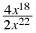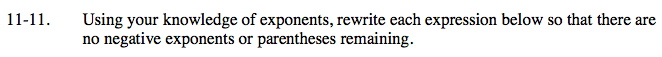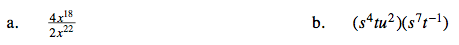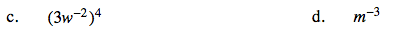Home > AC > Chapter 11 > Lesson 11.1.1 > Problem11-11

11-11.
1. Using your knowledge of exponents, rewrite each expression below so that there are no negative exponents or parentheses remaining. Homework Help ✎

1.2. (s4tu2)(s7t−1)

3. (3w−2)4

4. m−3Think about making ones and where any left over factors would remain.

$\frac{ 2 }{x^{4} }$

How many factors of s are multiplied?
How many of t?
How many of u?

s11u2Write 3w−2 multiplied four times then follow the same pattern as seen in part (b).

$\frac{ 81 }{w^{8} }$

Move the exponent and its base into the denominator
of a fraction to make the exponent positive.

$\frac{ 1 }{m^{3} }$